https://doi.org/10.5194/os-17-909-2021
https://doi.org/10.5194/os-17-909-2021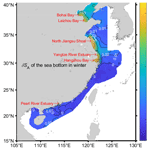# Estimating the Absolute Salinity of Chinese offshore waters using nutrients and inorganic carbon data

Fengying Ji, Rich Pawlowicz, and Xuejun Xiong
Abstract

In June 2009, the Intergovernmental Oceanographic Commission of UNESCO released The international thermodynamic equation of seawater – 2010 (TEOS-10 for short; IOC et al., 2010) to define, describe and calculate the thermodynamic properties of seawater. Compared to the Equation of State-1980 (EOS-80 for short), the most obvious change with TEOS-10 is the use of Absolute Salinity as salinity argument, replacing the Practical Salinity used in the oceanographic community for 30 years. Due to the lack of observational data, the applicability of the potentially increased accuracy in Absolute Salinity algorithms for coastal and semi-enclosed seas is not very clear to date. Here, we discuss the magnitude, distribution characteristics, and formation mechanism of Absolute Salinity and Absolute Salinity Anomaly in Chinese shelf waters, based on the Marine Integrated Investigation and Evaluation Project of the China Sea and other relevant data. The Absolute Salinity SA ranges from 0.1 to 34.66 g kg−1. Instead of silicate, the main composition anomaly in the open sea, CaCO3 originating from terrestrial input and re-dissolution of shelf sediment is most likely the main composition anomaly relative to SSW and the primary contributor to the Absolute Salinity Anomaly δSA. Finally, relevant suggestions are proposed for the accurate measurement and expression of Absolute Salinity of the China offshore waters.

Share
Dates
1 Introduction

Absolute Salinity, which is traditionally defined as the mass fraction of dissolved material in seawater, replaces Practical Salinity as the salinity argument in the TEOS-10 (IOC et al., 2010) seawater standard for the thermodynamic properties of seawater. This is because these thermodynamic properties are directly influenced by the mass of dissolved constituents, whereas Practical Salinity depends only on their conductivity. Since the relative amounts of different constituents change from place to place and from time to time, accounting for the biases that are introduced by these changes may be important. However, appropriate methods for frequent and regular measurements of the dissolved content directly in ocean studies are still a topic of research.

At present, the TEOS-10 Absolute Salinity of a seawater sample is obtained by adding the Absolute Salinity Anomaly δSA to Reference Salinity SR, in which SR is the mass fraction of dissolved material in a stoichiometric composition model (the Reference Composition or RC) of seawater, defined by Millero (2008), for which the reference material known as International Association for the Physical Sciences of the Ocean (IAPSO) Standard Seawater (SSW for short), is a good approximation and of the same conductivity as that of the sample. δSA is the mass fraction change caused by composition variations relative to RC. Three algorithms for calculating Absolute Salinity in the open ocean are provided in TEOS-10. The two that avoid a direct measurement either make assumptions about the dominant biogeochemical processes in the ocean that affect the Absolute Salinity Anomaly or rely on empirically determined correlations.

However, the applicability and accuracy of the TEOS-10 algorithms are still not very clear for estuaries and semi-enclosed oceanic basins where the relative compositions of the seawater may be different from that of the open ocean. Although there have only been very few direct measurements of conductivity and density in such areas (Millero, 1984; Feistel et al., 2010a), Pawlowicz (2015) used chemical-composition–conductivity–density modeling and climatological data to estimate the Absolute Salinity Anomaly near many rivers around the world, finding values of up to 1 order of magnitude higher than those extrapolated from the open ocean.

The coastal areas of China comprise one of the widest shallow seas in the world, with a large north–south span, numerous estuaries and bays, and a large amount of freshwater input from rivers. The relative composition of this coastal seawater may not only differ from that of the open ocean but also vary from place to place. However, the influence of relative composition variation on the Absolute Salinity in this area has never been systematically studied, although salinity measurement has played an important role in Chinese national ocean survey projects since 1957 (CSTPRC, 1964) and for metrological purposes a Chinese primary seawater standard has been developed (Li et al., 2016). Moreover, in any efforts to detect salinity variations associated with climate change variability in the Bohai and northern Yellow seas (Wu et al., 2004a, b; Xu, 2007; Lv, 2008; Song, 2009), Practical Salinity SP is still used as the simplicity of Absolute Salinity, and its change caused by the relative composition variation is ignored. That will raise obvious problems in the correct presentation of time series and/or transects that begin near the coast and end well offshore (Wright et al., 2011).

Therefore, in this paper we first clarify the definition, status, and application of TEOS-10 Absolute Salinity. Second, based on the measured data and related research results, we estimate the magnitude, temporal and spatial distribution characteristics, and formation mechanisms giving rise to Absolute Salinity Anomalies in Chinese coastal seawaters. Finally, based on the above results, we put forward relevant suggestions and future research directions for the accurate measurement and expression of Absolute Salinity of Chinese offshore seawaters.

2 Methods and data

## 2.1 Calculation of Absolute Salinity

The TEOS-10 Solution Absolute Salinity of seawater is essentially based on adding up the mass of solute in a seawater sample:

$\begin{array}{}\text{(1)}& {S}_{\mathrm{A}}^{\text{soln}}=\sum _{i=\mathrm{1}}^{{N}_{\mathrm{c}}}{M}_{i}{c}_{i},\end{array}$

where ci is the molar concentration of component i in seawater kg−1, Mi is the molar mass of the component, and Nc is the number of species of component in seawater. However, it is impractical to carry out a full chemical analysis for the seawater to get the ${S}_{\mathrm{A}}^{\text{soln}}$ regularly. The primary and most demanding purpose of oceanographic salinity measurements is the calculation of seawater density to estimate significant ocean currents driven by sometimes tiny horizontal pressure gradients. In TEOS-10, Absolute Salinity is instead defined so that the density of seawater can be accurately calculated by the following equation:

$\begin{array}{}\text{(2)}& \mathit{\rho }={f}_{\text{TEOS-10}}\left({S}_{\mathrm{A}},t,p\right),\end{array}$

where fTEOS-10 is a specified function. Therefore, SA is also called a Density Salinity.

Unfortunately, although for many purposes we can treat SA and ${S}_{\mathrm{A}}^{\text{soln}}$ interchangeably, at highest precisions ${S}_{\mathrm{A}}\ne {S}_{\mathrm{A}}^{\text{soln}}$ due to small changes in the relative composition of sea salt. In order to get SA at this highest precision, Millero (2008) first defines a stoichiometric composition model (the Reference Composition or RC), based on a reference material (IAPSO Standard Seawater), and specifies an algorithm to determine a consistent estimate of the mass fraction of dissolved material in a sample of arbitrary salinity with the RC. This estimate is based on the widely used Practical Salinity SP (UNESCO, 1981):

$\begin{array}{}\text{(3)}& {S}_{\mathrm{R}}={u}_{\text{PS}}\cdot {S}_{\mathrm{P}},\phantom{\rule{1em}{0ex}}\mathrm{2}<{S}_{\mathrm{P}}<\mathrm{42}.\end{array}$

In Eq. (3), the factor uPS between the Reference Salinity of Standard Seawater and the Practical Salinity is (35.16504/35) g kg−1 and is not equal to 1 mainly because an evaporative technique used by Sørensen in 1900 (Forch et al., 1902) led to the loss of some volatile components of dissolved material.

General seawater can be regarded as the mixture of Standard Seawater concentrated/diluted with pure water and a small amount of other components. The calculation formula of Absolute Salinity from Reference Salinity requires the addition of a correction, the Absolute Salinity Anomaly δSA:

$\begin{array}{}\text{(4)}& {S}_{\mathrm{A}}={S}_{\mathrm{R}}+\mathit{\delta }{S}_{\mathrm{A}}.\end{array}$

At present there are three methods for determining the Absolute Salinity Anomaly δSA. First, it can be obtained by comparisons with direct density measurements performed in the laboratory (Millero et al., 2008; Wright et al., 2011). According to the density difference $\mathit{\rho }={\mathit{\rho }}^{\text{lab}}-\mathit{\rho }\left({S}_{\mathrm{R}},\mathrm{25}\phantom{\rule{0.125em}{0ex}}{}^{\circ }\mathrm{C},\mathrm{0}\phantom{\rule{0.125em}{0ex}}\mathrm{dbar}\right)$ and the haline contraction coefficient, which is 0.7519 for SSW, δSA is determined by

This procedure is useful for laboratory studies or in situations where ocean water can be obtained from sampling bottles retrieved from certain depths for subsequent laboratory measurements of density.

Second, it can be estimated using a correlation equation whether chemical measurements of the most variable seawater constituents in the open ocean (carbonate system and macro-nutrients) are also available (Pawlowicz et al., 2011; IOC et al., 2010).

$\begin{array}{}\text{(6)}& \begin{array}{rl}& \mathit{\delta }{S}_{\mathrm{A}}^{\text{dens}}/\left(\mathrm{mg}\phantom{\rule{0.125em}{0ex}}{\mathrm{kg}}^{-\mathrm{1}}\right)=\phantom{\rule{0.25em}{0ex}}\mathrm{55.6}×\mathrm{\Delta }\left[\text{NTA}\right]+\mathrm{4.7}\\ & ×\mathrm{\Delta }\left[\text{NDIC}\right]+\mathrm{38.9}×\left[{\mathrm{NO}}_{\mathrm{3}}^{-}\right]+\mathrm{50.7}×\left[{\mathrm{S}}_{i}\left(\mathrm{OH}{\right)}_{\mathrm{4}}\right]\end{array}\end{array}$

The units of each component on the right are all millimole per kilogram, $\mathrm{\Delta }\left[\text{NTA}\right]=\text{TA}-\mathrm{2.3}×{S}_{\mathrm{P}}/\mathrm{35}$ is the standardized change in Total Alkalinity (TA), and $\mathrm{\Delta }\left[\text{NDIC}\right]=\text{DIC}-\mathrm{2.08}×{S}_{\mathrm{P}}/\mathrm{35}$ is the standardized change in total Dissolved Inorganic Carbon (DIC). Note that the coefficients of this model are calculated using a numerical model for chemical interactions (Pawlowicz, 2008, 2010; Pawlowicz et al., 2011), which performed well against lab studies and were shown to have reasonable accuracy for seawater samples by Wooseley et al. (2014). An important aspect of this modeling is that, in order to maintain a charge balance in the dissolved constituents, it was assumed that calcium concentrations also changed according to

$\begin{array}{}\text{(7)}& \mathrm{\Delta }\left[\text{NTA}\right]=\mathrm{2}\mathrm{\Delta }\mathrm{N}\left[{\mathrm{Ca}}^{\mathrm{2}+}\right]-\mathrm{\Delta }\left[{\mathrm{NO}}_{\mathrm{3}}^{-}\right],\end{array}$

in which $\mathrm{\Delta }\mathrm{N}\left[{\mathrm{Ca}}^{\mathrm{2}+}\right]={\mathrm{Ca}}^{\mathrm{2}+}-\mathrm{10656.6}\cdot {S}_{\mathrm{P}}/\mathrm{35}/\left(\mathrm{µ}\mathrm{mol}\phantom{\rule{0.125em}{0ex}}{\mathrm{kg}}^{-\mathrm{1}}\right)$ and Ca2+ and SP are the measured value of Ca2+ and Practical Salinity of seawater, respectively. Calcium was chosen to balance charge since it is (a) not usually measured but (b) it is known to vary in its relative composition by a few percent in the open ocean. However, the accuracy of this relationship is not known.

Third, Absolute Salinity Anomaly δSA can be found from a global δSA climatology created by McDougall et al. (2012). Due to the lack of seawater component data, McDougall et al. (2012) carried out regression calculation on the Practical Salinity, density, and silicate concentration data of 811 seawater samples worldwide and found that δSA can be directly related to Si(OH)4:

$\begin{array}{}\text{(8)}& \begin{array}{rl}& \mathit{\delta }{S}_{\mathrm{A}}/\left(\mathrm{g}\phantom{\rule{0.125em}{0ex}}{\mathrm{kg}}^{-\mathrm{1}}\right)\phantom{\rule{0.25em}{0ex}}=\left({S}_{\mathrm{A}}-{S}_{\mathrm{R}}\right)/\left(\mathrm{g}\phantom{\rule{0.125em}{0ex}}{\mathrm{kg}}^{-\mathrm{1}}\right)\\ & \phantom{\rule{0.25em}{0ex}}=\mathrm{98.24}\left({\mathrm{S}}_{i}\left(\mathrm{OH}{\right)}_{\mathrm{4}}/\left(\mathrm{mol}\phantom{\rule{0.125em}{0ex}}{\mathrm{kg}}^{-\mathrm{1}}\right)\right),\end{array}\end{array}$

although for further work the numerical coefficient was tuned for specific ocean basins. Taking the effects of evaporation and rainfall on ocean salinity into consideration, Eq. (8) can be simplified as

$\begin{array}{}\text{(9)}& \mathit{\delta }{S}_{\mathrm{A}}={R}^{\mathit{\delta }}{S}_{\mathrm{R}}\phantom{\rule{1em}{0ex}}\text{(except the Baltic Sea)},\end{array}$

in which ${R}^{\mathit{\delta }}=\mathit{\delta }{S}_{\mathrm{A}}^{\text{atlas}}/{S}_{\mathrm{R}}^{\text{atlas}}$; both the ${S}_{\mathrm{R}}^{\text{atlas}}$ and $\mathit{\delta }{S}_{\mathrm{A}}^{\text{atlas}}$ are from the Gouretski and Koltermann (2004) hydrographic atlas.

$\begin{array}{}\text{(10)}& {S}_{\mathrm{A}}={u}_{\text{PS}}{S}_{\mathrm{P}}\left(\mathrm{1}+{R}^{\mathit{\delta }}\right)=\frac{\mathrm{35.16504}/\left(\mathrm{g}\phantom{\rule{0.125em}{0ex}}{\mathrm{kg}}^{-\mathrm{1}}\right)}{\mathrm{35}}{S}_{\mathrm{P}}\left(\mathrm{1}+{R}^{\mathit{\delta }}\right)\end{array}$

Equation (10) is adopted in the official Gibbs SeaWater Oceanographic Toolbox (available from http://www.teos-10.org, last access: 8 June 2021, McDougall and Barker, 2011) to calculate that δSA with uncertainty in the ocean is less than 0.0047 g kg−1. For the semi-enclosed Baltic Sea, Feistel et al. (2010a) have fitted an empirical formula for calculating δSA, which is mainly due to rivers bringing material of anomalous composition into the Baltic Sea, and this formula has also been incorporated into the Gibbs SeaWater (GSW for short) algorithm library.

In the work described here we compare the latter two methods.

## 2.2 Observation data

The near-synchronous oceanographic and ocean chemical data used here are from 1480 stations covering Chinese offshore waters that were set up for the Marine Integrated Investigation and Evaluation Project of the China Sea conducted by the State Oceanic Administration of China (Xiong, 2012; Ji, 2016), as shown in Fig. 1. At these sites, surface, 10 m, 30 m and bottom values for nutrients, as well as TA and pH, are available for the four seasons of spring (April–June), summer (July–September), autumn (October–December), and winter (January–March) of 2006 to 2007. Since in situ observation of DIC is missing in this project, it is derived from pH and TA data using the CO2SYS software released by the Department of Ecology of Washington State, USA, based on the carbonate equilibrium (Lewis and Wallace, 1998).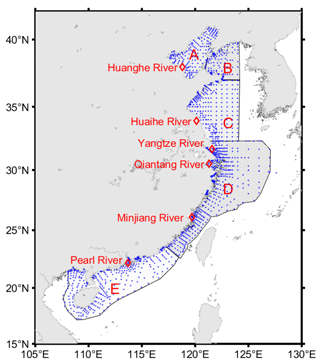Figure 1The geographical distribution map of sampling stations. The blue dots are the observation stations of the Marine Integrated Investigation and Evaluation Project of the China Sea. “A” is the Bohai Sea, “B” is the northern Yellow Sea, “C” is the southern Yellow Sea, “D” is the East China Sea, and “E” is the South China Sea.

3 Results

## 3.1 Reference Salinity SR of the China offshore seawater

The first step in determining Absolute Salinity is to estimate the Reference Salinity based on the Practical Salinity. Because the standard PSS-78 algorithm for Practical Salinity is only valid in the range $\mathrm{2}<{S}_{\mathrm{P}}<\mathrm{42}$, values for samples in the mouth of the Yangtze River, Qiantang River, and Pearl River (labeled in Fig. 1) whose SP values less than 2 are recalculated with a modified form of the Hill et al. (1986) formula based on the in situ conductivity, temperature, and pressure. Then Eq. (3) is used to get SR.

Based on our observations (Fig. 1), the Reference Salinity SR of Chinese offshore seawater diluted by low-salinity river runoff ranges from 0.01 to 34.66 g kg−1. The extreme minimum SR of 0.01 g kg−1 appears in the south branch of Yangtze River in the summer of 2006, and the maximum of 34.66 g kg−1 appears in the path of the Kuroshio Current (Fig. 2). Low salinities are also seen in the Pearl River estuary and to a lesser degree in shallow areas of the southern Yellow Sea, as well as near a few other river mouths.

## 3.2 Absolute Salinity Anomaly δSA of Chinese offshore waters

Using Eq. (6), the estimated δSA of Chinese offshore waters ranges from −0.05 to 0.28 g kg−1 (Fig. 3). The largest Absolute Salinity Anomalies are 1 order higher than those of the open ocean. As much as 90 % of the calculated δSA arises from the Δ[NTA] term in Eq. (6), so that areas with high δSA also have high Δ[NTA] (Fig. 4). The largest δSA values appear in the Yangtze River estuary, Hangzhou Bay, Laizhou Bay, Bohai Bay, North Jiangsu Shoal, and the Pearl River estuary. Hangzhou Bay, which is adjacent to the Yangtze River estuary, has continuously transported water from the Yangtze River estuary due to its current and tidal characteristics (Yuan, 2009) and has almost the same water composition as the Yangtze River estuary. Thus, in this paper, the waters in the Yangtze River estuary and Hangzhou Bay are analyzed as a single water mass. The δSA values in the above coastal regions, which are often in excess of 0.05 g kg−1, are given in Table 1.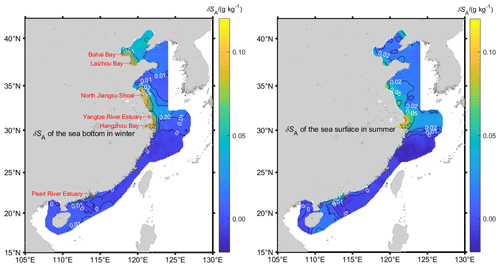Figure 3δSA isoclines of Chinese offshore seawater. Hatched areas in the left figure represent the areas where δSA is more than 0.05 g kg−1.

Table 1δSA in different coastal regions hatched in Fig. 3. Units are milligrams per kilogram.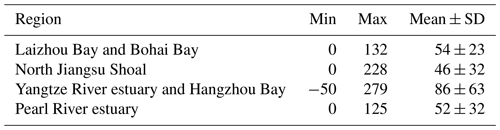The maximum δSA of 0.28 g kg−1 appears at the sea surface of the Yangtze River estuary and in Hangzhou Bay in summer. As China's largest runoff into the sea, the Yangtze River is rich in nutrients from land. At its entrance to the sea, the silicate concentration exceeds 100 µmol kg−1, Δ[NTA] is larger than 1 mmol kg−1, and the δSA is greater than 0.1 g kg−1 all year round, but these nutrient concentrations decrease rapidly away from the entrance. Δ[NTA] is the primary contributor to δSA. The surface coverage of the 0.05 g kg−1 isocline varies with seasons and depths and reaches a maximum in summer but with little variation in other seasons. In this region, 54 % and 26 % of negative δSA appear in spring and winter, respectively, which also mainly arises from Δ[NTA].

In the northern North Jiangsu Shoal, the maximum δSA of 0.23 g kg−1 appears in the bottom layer in winter. Centered at 33.4 N and 121 E, many points have a δSA greater than 0.05 g kg−1 all year round, which gradually decreases from the coast to the offshore. The δSA of the bottom layer is higher than that of the surface layer in a dry season (spring and winter) but smaller in a flood (summer and autumn) season, in which more terrestrial input is brought by Huai River system.

The largest δSA of 0.20 g kg−1 in the Bohai Sea appears at the bottom of Laizhou Bay in winter, and seasonal characteristics are basically the same as the North Jiangsu Shoal, although in summer more terrestrial material is input by the Yellow River. As the Bohai Sea is a semi-enclosed shallow sea with lower exchange with the open ocean, the δSA in the whole Bohai Sea is always larger than 0.02 g kg−1 and the δSA difference between the bottom and the surface within the same season is not as significant as its seasonal variation in the area.

A δSA of greater than 0.05 g kg−1 also occurs at the mouth of the Pearl River and Min River in summer, but values are less than 0.02 g kg−1 in other seasons. However, these values are seen within the estuary with very little presence on the shelf. In the remaining areas, the magnitude of δSA is below 0.005 g kg−1, which is about the same as the magnitude of the statistical uncertainty of the Absolute Salinity Anomaly in the open ocean and so is essentially zero.

Although we have used Eq. (6), which is meant for seawater of relatively high salinity, to estimate the Absolute Salinity Anomaly near river mouths where the salinity is far smaller, a more complex calculation of the δSA, based on a full chemical analysis of river water composition, was plotted for some of these rivers (the Yangtze, the Pearl and Min rivers) in Pawlowicz (2015). The values calculated in that work are consistent with those found here (Table 2).

Table 2δSA in some rivers as estimated in this paper, compared with values estimated using a more complete theory in Pawlowicz (2015). Units are milligrams per kilogram.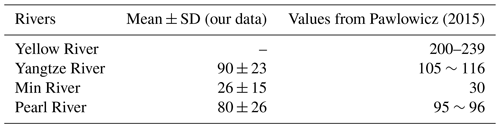## 3.3 Parameterization of the Absolute Salinity of the China offshore waters

Although the Absolute Salinity Anomalies within rivers are always non-zero, the Absolute Salinity Anomaly is significantly non-zero in only four areas along the Chinese coast and river mouths (hatched areas in Fig. 3). They are occupied by different coastal water masses (Xiong, 2012), and the Absolute Salinities Anomalies in each can be parameterized separately.

China offshore seawater is a mixture of the Kuroshio water originating from the North Equatorial Current and the runoff into the sea. The Absolute Salinity Anomaly in Pacific surface waters in any case is generally small; it is the deeper waters that have (relatively) large Absolute Salinity Anomalies arising from remineralization in the subsurface branch of the ocean's overturning circulation. In this paper, we ignore the relative composition difference between the Kuroshio and SSW for now. Following Feistel et al. (2010b), these four water masses are regarded as the mixture of Standard Seawater that has standard-ocean salinity, with the local coastal water which contains unknown amounts of unknown solute. The related regression lines of the four water masses between Absolute Salinity Anomaly and the Reference Salinity can be computed from the samples with salinity SR>2g kg−1, in which the seawater endpoints are chosen to be SSW with a δSA of zero, as shown in Eq. (11) and Fig. 5.

$\begin{array}{l}{S}_{\mathrm{A}}-{S}_{\mathrm{R}}=\left[\begin{array}{c}\mathrm{412}\\ \mathrm{236}\\ \mathrm{150}\\ \mathrm{107}\end{array}\right]/\left(\mathrm{mg}\phantom{\rule{0.125em}{0ex}}{\mathrm{kg}}^{-\mathrm{1}}\right)\cdot \left(\mathrm{1}-\frac{{S}_{\mathrm{R}}}{{S}_{\text{SO}}}\right),\\ \text{(11)}& \left[\begin{array}{c}\text{Laizhou Bay and Bohai Bay}\\ \text{North Jiangsu Shoal}\\ \text{Yangtze River estuary and Hangzhou Bay}\\ \text{Pearl River estuary}\end{array}\right]\end{array}$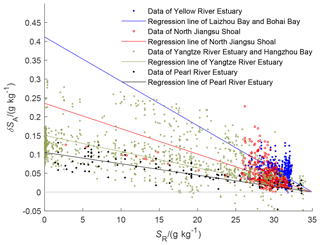Figure 5The Absolute Salinity Anomaly of the four regions of China offshore waters as a function of their Reference Salinity.

The linear correlation between Absolute Salinity Anomaly and SR in the Pearl River estuary is the strongest among the four regions, which shows that the mixture between the coastal seawater and that of the open ocean is relatively conservative. There are many measurements over all salinities for the Yangtze River water. The strong scatter visible in Fig. 5 at low salinities is likely due to the rich (and highly variable) nutrient loading brought by Yangtze River draining from land.

The regressions for the two northernmost areas are less precise, as the oceanographic sampling pattern does not enter into the rivers and measured salinities are larger than 25 g kg−1. The fitted curves are somewhat steeper. Note that Pawlowicz (2015) also finds that Absolute Salinity Anomalies in the Yellow River of about 0.2 g kg−1 are also higher than in the other rivers (Table 2), although not as high as our fits in Fig. 5 suggest. The fit for the North Jiangsu Shoal region is heavily influenced by many high values when salinities are between 20 and 25 g kg−1 and lies somewhat above a smaller number of values spread over lower salinities.

It can be seen from Fig. 5 that the relative composition anomalies decrease from north to south. The exchange of coastal waters with the open-ocean waters increases gradually from the northernmost (and somewhat enclosed) Bohai Sea estuary to the southernmost Pearl River area, which is open to the South China Sea.

## 3.4 Relative composition anomaly of China offshore seawater

In Eq. (6), the coefficients are determined by fitting to the results of more complete calculations that assume changes to Ca2+ to maintain a charge balance according to Eq. (7). We cannot directly check the accuracy of this assumption. However, Ca2+ was directly measured from samples in 13 cruises from April 2011 to February 2012 (Qi, 2013). Although these measurements do not occur at the same time as our larger dataset, we can group these measurements in the same regions (labeled in Fig. 1) in which we find large Absolute Salinity Anomalies. Then, we find that the ΔN[Ca2+], $\mathrm{\Delta }\left[{\mathrm{NO}}_{\mathrm{3}}^{-}\right]$, and Δ[NTA] (first column) values from our dataset (Table 3) are approximately consistent with Eq. (7).

Table 3The mean value of Δ[NTA], $\mathrm{\Delta }\left[{\mathrm{NO}}_{\mathrm{3}}^{-}\right]$, Δ[NTA], and $\mathrm{\Delta }\mathrm{N}\left[{\mathrm{Ca}}^{\mathrm{2}+}{\right]}^{\ast }$ are given in different areas (marked in Fig. 1). Values obtained from Qi (2013) are labeled with “”. Units are micromole per kilogram.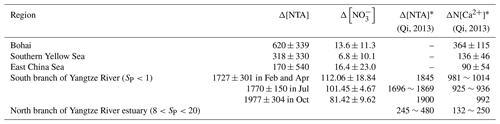The other nutrient of phosphate is not considered in the calculation, for its concentrations range from 0 to 0.01 mmol kg−1 in the existing observation, which is much smaller than those items in Eq. (7) above, and its effect is negligible. In this case, $\mathrm{\Delta }\left[{\mathrm{NO}}_{\mathrm{3}}^{-}\right]$ is mostly negligible and ΔN[Ca2+] is about 43 %∼58 % of Δ[NTA], in the Bohai Sear, southern Yellow Sea, East China Sea, and the Yangtze River.

The importation of Ca2+ and the carbon system suggest that the major source of Absolute Salinity Anomalies in shelf areas is the high CaCO3 content of rivers. This is consistent with Absolute Salinity Anomalies in the Baltic Sea, which were found to be mostly related to the calcium carbonate input from rivers (Feistel et al., 2010a). These rivers would be the Yangtze, Yellow River, and Huai rivers. The importation fluxes of Ca2+ into the sea from the Yellow River and the Yangtze River are 3.6×1010 and 6.5×1011mol yr−1, respectively, in 2011 (Qi, 2013). In addition, there may be re-dissolution of sediments in the Yellow River estuary and North Jiangsu Shoal. Due to the accumulation of materials entering the sea from the old Yellow River and the ancient Yangtze River, the CaCO3 concentration of surface sediments on the seafloor of the North Jiangsu Shoal ranges from 2.8 % to 10.5 % (Qin et al., 1989; Yang and Youn, 2007). The ΔNDIC of the southern Yellow Sea near China has always been high; even when strong biological activity in spring reduces the surface Δ[NTA], the sediment of particulate inorganic carbon will resuspend and maintain the high level of dissolved CaCO3 of seawater through the solid–liquid balance (Hong, 2012; Zhang et al., 1995).

## 3.5 Contrast to the δSA calculated by GSW

Using the GSW function library and the corresponding climatological silicate and Practical Salinity data, the calculated δSA of China offshore waters ranges from 0 to 0.002 g kg−1. This is 2 orders of magnitude less than the values calculated in Sect. 3.2. The spatial distribution characteristics are also significantly different. These differences mainly come from the following aspects:

1. Instead of silicate, CaCO3 is most likely the main relative composition anomaly of China offshore seawater and the primary contributor to the δSA, where it is greater than 0.05 g kg−1.

2. High silicate concentrations (up to 100 µmol kg−1) do appear in Chinese coastal seawaters from the effects of rivers (Fig. 6), but these do not appear in the global silicate climatology used for the GSW calculations. However, even if they did, in these places Δ[NTA] is even larger, so that the effects of this coastal silicate on the Absolute Salinity Anomaly are small.

In the remaining areas, the silicate concentration is less than 20 µmol kg−1, as shown in Fig. 6 at a 95 % degree of confidence; the difference between the observation and the GSW climatological dataset is [5.46, 6.21] µmol kg−1, which does not change much with the seasons. It can be indicated that the GSW climatological dataset basically reflects the distribution characteristics of silicate in these areas.

4 Conclusion and analysis

The proposal and implementation of the concept of SA in TEOS-10 are meant to accurately quantify the total mass of inorganic substance dissolved in seawater, to ensure that the density and related quantities are accurately represented by the Gibbs function for seawater, and to correct errors caused by measuring the properties of seawater such as chloride and conductivity to get the salinity. In this paper, based on observations and calculations, the magnitude, distribution characteristics of Absolute Salinity in China offshore waters are described as follows:

1. The Absolute Salinity SA ranges from 0.1 to 34.66 g kg−1, in which SR ranges from 0.01 to 34.66 g kg−1, and the Absolute Salinity Anomaly δSA ranges from −0.05 to 0.28 g kg−1; this is an order of magnitude larger than the largest values in the open ocean.

2. The largest δSA values are located in four distinct regions: the Yangtze River mouth/Hangzhou Bay, the North Jiangsu Shoal, the Bohai Sea, and the Pearl River mouth, all of which are areas where the Δ[NTA] is high.

3. Instead of silicate, CaCO3 is most likely the main composition anomaly relative to SSW and the primary contributor to the δSA in the above four areas.

4. Under the combined effects of different water system dynamics, terrestrial input, marine biological activities, and re-dissolution of marine sediments, the δSA values in China offshore waters' seasonal variations are obvious, and the maximum can be as high as 0.05 g kg−1; the difference between the surface layer and the bottom layer is also up to 0.1 g kg−1.

With the observations available, this paper only lists the magnitude and distribution characteristics of δSA in China offshore waters from 2006 to 2007, although it is likely that similar features will occur in other years. At present, we have collated the long-term series of seawater composition data to continue the study on δSA changes and get an empirical formula to calculate δSA.

The current research is only based on the existing seawater composition data, and the exact influence of other changes to composition is still not very clear. To verify these findings, a complete chemical analysis and/or direct measurements of seawater density would be useful in the estuaries of the Yangtze River, Qiantang River, Pearl River, Min River, and the semi-enclosed Bohai Sea.

Code availability

MATLAB-version of CO2SYS is available at https://github.com/jamesorr/CO2SYS-MATLAB (Lewis and Wallace, 2021).

Data availability

The research data used in this manuscript have not been publicly available yet because the investigators are still conducting relevant research based on these massive data. At present, the relevant atlas and research reports have been officially published and listed in the references list: (1) State Ocean Administration of China: China Offshore Atlas – Ocean Chemistry, ocean press, Beijing, 2016. (2) State Ocean Administration of China: China Offshore Atlas – Oceanography, ocean press, Beijing, 2016. (3) Xiong, X. J.: China Regional Oceanography and Marine Meteorology,Ocean Press, Beijing, 2012. (4) Ji, W. D.: China Offshore – Ocean Chemistry, Ocean Press, Beijing, 2016.

Author contributions

FJ was responsible for method design and implementation, writing original drafts, verifying editorial opinions, and reviewing and editing. RP revised a few key theories in the draft, provided the model data to verify the result in the draft, and was involved in review and editing. XX was the project administrator and was involved in topic selection and review and editing.

Competing interests

The authors declare that they have no conflict of interest.

Acknowledgements

The authors express their gratitude to Guo Xianghui and Wang Haili from Xiamen University for providing useful suggestions for marine chemical data evaluation.

Financial support

This research has been supported by the National Natural Science Foundation of China (grant no. 41406024) and the National Key Research and Development Program of China (grant no. 2017YFA0604904).

Review statement

This paper was edited by Trevor McDougall and reviewed by Paul Barker and two anonymous referees.

References

Commission of Science and Technology of the People's Republic of China: Report of the National Comprehensive Marine Survey, Volume I, 1964.

Feistel, R., Marion, G. M., Pawlowicz, R., and Wright, D. G.: Thermophysical property anomalies of Baltic seawater, Ocean Sci., 6, 949–981, https://doi.org/10.5194/os-6-949-2010, 2010a.

Feistel, R., Weinreben, S., Wolf, H., Seitz, S., Spitzer, P., Adel, B., Nausch, G., Schneider, B., and Wright, D. G.: Density and Absolute Salinity of the Baltic Sea 2006–2009, Ocean Sci., 6, 3–24, https://doi.org/10.5194/os-6-3-2010, 2010b.

Gouretski, V. V. and Koltermann, K. P.: WOCE Global Hydrographic Climatology, Berichte des Bundesamtes fur Seeschifffahrt und Hydrographie, Tech. Rep., 35, 49, 2004.

Hill, K. D., Dauphinee, T. M., and Woods, D. J.: The extension of the Practical Salinity Scale 1978 to low salinities, IEEE J. Ocean. Eng., OE-11, 109–112, 1986.

Hong, H. S.: China Regional Oceanography – Chemical Oceanography, Ocean Press, Beijing, 2012.

IOC, SCOR, IAPSO: The International Thermodynamic Equation of Seawater – 2010: Calculation and Use of Thermodynamic Properties. Manual and Guides No. 56, Intergovernmental Oceanographic Commission, UNESCO (English), 2010.

Ji, W. D.: China Offshore – Ocean Chemistry, Ocean Press, Beijing, 2016.

Lewis, E. and Wallace, D. W. R.: Program developed for CO2 system calculations, ORNL/CDIAC-105, Oak Ridge National Laboratory, 1998.

Lewis, E. and Wallace, D. W. R.: Program Developed for CO2 System Calculations, Github [Dataset], available at: https://github.com/jamesorr/CO2SYS-MATLAB, last access: 8 June 2021.

Li, Y., Luo, Y., Kang, Y., Yu, T., Wang, A., and Zhang, C.: Chinese primary standard seawater: stability checks and comparisons with IAPSO Standard Seawater, Deep-Sea Res., 113, 101–106, 2016.

Lv, C. L.: Analysis of decadal variability of salinity field and its influence to circulation in Bohai and northern Yellow Sea, The Master Degree Dissertation of China Ocean University, 2008.

McDougall, T. J. and Barker, P. M.: Getting started with TEOS-10 and the Gibbs Seawater (GSW) Oceanographic Toolbox, 28 pp., SCOR/IAPSO WG127, ISBN 978-0-646-55621-5, 2011.

McDougall, T. J., Jackett, D. R., Millero, F. J., Pawlowicz, R., and Barker, P. M.: A global algorithm for estimating Absolute Salinity, Ocean Sci., 8, 1123–1134, https://doi.org/10.5194/os-8-1123-2012, 2012.

Millero, F. J.: The conductivity–density–salinity–chlorinity relationships for estuarine waters, Limnol. Oceanogr., 29, 1317–1321, 1984.

Millero, F. J., Feistel, R., Wright, D. G., and McDougall, T. J.: The composition of Standard Seawater and the definition of the Reference-Composition Salinity Scale, Deep-Sea Res. Pt. I, 55, 50–72, 2008.

Pawlowicz, R.: Calculating the conductivity of natural waters, Limnol. Oceanogr.: Methods, 6, 489–501, 2008.

Pawlowicz, R.: A model for predicting changes in the electrical conductivity, practical salinity, and absolute salinity of seawater due to variations in relative chemical composition, Ocean Sci., 6, 361–378, https://doi.org/10.5194/os-6-361-2010, 2010.

Pawlowicz, R.: The Absolute Salinity of seawater diluted by river water, Deep-Sea Res. Pt. I, 101 71–79, 2015.

Pawlowicz, R., Wright, D. G., and Millero, F. J.: The effects of biogeochemical processes on oceanic conductivity/salinity/density relationships and the characterization of real seawater, Ocean Sci., 7, 363–387, https://doi.org/10.5194/os-7-363-2011, 2011.

Qi, D.: Dissolved calcium in the Yangtze River Estuary and China Offshore, The Master Degree Dissertation of Xiamen University, 2013.

Qin, Y. S., Zhao, Y. Y., and Chen L. R.: Huanghai Geology, Science Press, Beijing, 1989.

Song, W. P.: The analysis of the structure of T-S and the current characteristics in Bohai Sea during winter and summer, The Master Degree Dissertation of China Ocean University, 2009.

UNESCO: The Practical Salinity Scale 1978 and the International Equation of State of Seawater 1980, UNESCO Technical Papers in Marine Science, 36, 25 pp., 1981.

Yuan, J. Z.: Study on water exchange characteristics between Yangtze Estuary and Hangzhou Bay, The Third Yangtze River Forum Proceedings (in Chinese), 183–191, 2009.

Woosley, R. J., Huang, F., and Millero, F. J.: Estimating absolute salinity (SA) in the world's oceans using density and composition, Deep Sea Res. Pt. I, 93, 14–20, 2014.

Wright, D. G., Pawlowicz, R., McDougall, T. J., Feistel, R., and Marion, G. M.: Absolute Salinity, “Density Salinity” and the Reference-Composition Salinity Scale: present and future use in the seawater standard TEOS-10, Ocean Sci., 7, 1–26, https://doi.org/10.5194/os-7-1-2011, 2011.

Wu, D. X., Mu, L., Li, Q., Bao, X.W, and Wan, X. Q.: Characteristics of long-term change and its possible dominant factors in Bohai Sea, Progress in Nature Science, 14, 191–195, 2004a.

Wu, D. X., Wan, X.Q, Bao, X.W, Mu, L., and Lan, J.: Comparison of temperature-sallinity field and circulation Structure in the Summer between 1958 and 2000, Chinese Sci. Bull., 49, 287–292, 2004b.

Xiong, X. J.: China Regional Oceanography and Marine Meteorology, Ocean Press, Beijing, 2012.

Xu, J. L.: The variation characters and formation mechanism of salinity in the Bohai Sea, The Master Degree Dissertation of China Ocean University, 2007.

Yang, S. Y. and Youn J. S.: Geochemical compositions and provenance discrimination of the central south Yellow Sea sediments, Mar. Geol., 243, 229–241, 2007.

Zhang, J., Huang, W. W., Létolle, R., and Jusserand, C.: Major element chemistry of the Huanghe River (Yellow River), China-weathering processes and chemical fluxes, J. Hydrol., 168, 173–203, 1995.